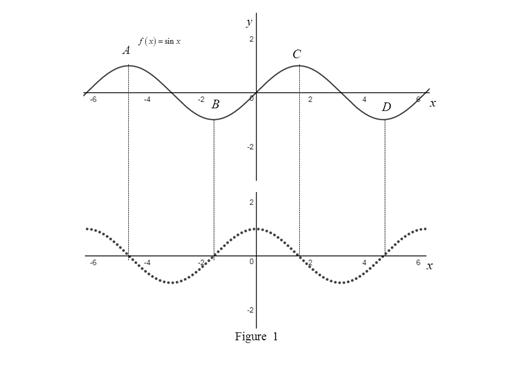# The graph of f ( x ) and then f ′ ( x ) below it and guess the formula for f ′ ( x ) .### Single Variable Calculus: Concepts...

4th Edition
James Stewart
Publisher: Cengage Learning
ISBN: 9781337687805### Single Variable Calculus: Concepts...

4th Edition
James Stewart
Publisher: Cengage Learning
ISBN: 9781337687805

#### Solutions

Chapter 2.7, Problem 14E
To determine

## To sketch: The graph of f(x) and then f′(x) below it and guess the formula for f′(x).

Expert Solution

The formula for f(x) from its graph is f(x)=cosx.

### Explanation of Solution

The given function is f(x)=sinx.

Recall the fact that, the derivative of a function at x=a is zero if the function has horizontal tangent at the point x=a.

From the graph, it is observed that the graph has a horizontal tangent at three points. Let the points be A, B, C and D. At these points, the derivative will be zero.

The AB section of the graph has negative slope which implies that the derivative will be negative in the section.

The BC section of the graph has positive slope which implies that the derivative will be positive in the section.

The CD section of the graph has negative slope which implies that the derivative will be negative in the section.

Graph:

Use the online graphing calculator to draw the graph of f(x)=sinx and use the above information to trace the graph of f(x) as shown below in Figure 1.Observation:

From the Figure 1, it is observed that the graph of the derivative function seems to be the cosine function.

Thus, the formula of the derivative function is, f(x)=cosx.

### Have a homework question?

Subscribe to bartleby learn! Ask subject matter experts 30 homework questions each month. Plus, you’ll have access to millions of step-by-step textbook answers!